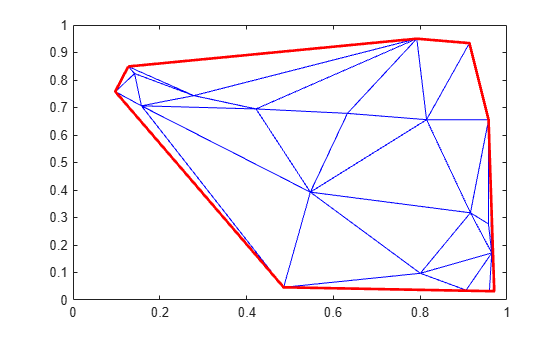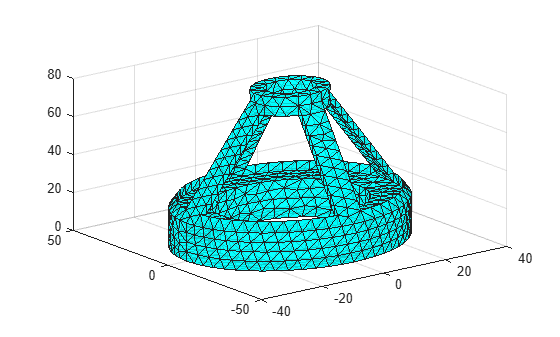freeBoundary

Free boundary facets

Description

example

F = freeBoundary(TR) returns the free boundary facets of the triangles or tetrahedra in TR. A facet in TR is on the free boundary if it is referenced by only one triangle or tetrahedron.

example

[F,P] = freeBoundary(TR) also returns a matrix containing the vertices of the free boundary facets.

Examples

collapse all

You can use the freeBoundary function to highlight the outer edges of a 2-D Delaunay triangulation.

Create a triangulation from a 2-D set of points.

rng('default')
x = rand([20,1]);
y = rand([20,1]);
TR = delaunayTriangulation(x,y);

Find the free boundary edges.

F = freeBoundary(TR);

Plot the triangulation and highlight the free boundary edges in red.

triplot(TR)
hold on
plot(x(F),y(F),'-r','LineWidth',2)You can use the freeBoundary function to extract the facets of a 3-D triangulation that cover the surface of an object.

TR = triangulation(tet,X);

Compute the boundary triangulation.

[F,P] = freeBoundary(TR);

Plot the boundary triangulation.

trisurf(F,P(:,1),P(:,2),P(:,3), ...
'FaceColor','cyan','FaceAlpha',0.8);Input Arguments

collapse all

Triangulation representation, specified as a scalar triangulation or delaunayTriangulation object.

Data Types: triangulation | delaunayTriangulation

Output Arguments

collapse all

Triangulation connectivity list, returned as a matrix.

If you call freeBoundary with one output argument, then the elements of F are row numbers in the Points property of the input triangulation. If you call freeBoundary with two output arguments, then the elements of F are row numbers in P.

Data Types: double

Free boundary points, returned as a matrix containing the coordinates of the vertices of the free boundary facets. Each row of P contains the coordinates of a vertex.

Data Types: double